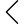90 products found
• s
• m
• l
• xl
LPU CMU Tanktop
\$143.65
• s
• m
• l
• xl
LPU CMU Tanktop
\$118.15
• s
• m
• l
• xl
LPU CMU Tanktop
\$143.65
• s
• m
• l
• xl
LPU CMU Tanktop
\$143.65
• s
• m
• l
• xl
• s
• m
• l
• xl
• s
• m
• l
• xl
• s
• m
• l
• xl
• s
• m
• l
• xl
• s
• m
• l
• xl
• s
• m
• l
• xl
• s
• m
• l
• xl
• s
• m
• l
• xl
• s
• m
• l
• xl
• s
• m
• l
• xl
• s
• m
• l
• xl
LPU CMU T-shirt
Sold out
• s
• m
• l
• xl
LPU CMU T-shirt
Sold out
• s
• m
• l
• xl
• s-m
• x-xl
• s
• m
• l
• xl
• s
• m
• l
• xl
• s
• m
• l
• xl
• s
• m
• l
• xl
LPU CMU T-shirt
\$149.00 \$126.65
• s
• m
• l
• xl
• s
• m
• l
• xl
• s
• m
• l
• xl
• s
• m
• l
• xl
• s
• m
• l
• xl
• s
• m
• l
• xl
• s
• m
• l
• xl
• s
• m
• l
• xl
• s
• m
• l
• xl
• s
• m
• l
• xl
• s
• m
• l
• xl
• s
• m
• l
• xl
• s
• m
• l
• xl
• s
• m
• l
• xl
• s
• m
• l
• xl
GP printed t-shirts
\$129.00 \$109.65
• s
• m
• l
• xl
• s
• m
• l
• xl
•• 1
• 2
• 3